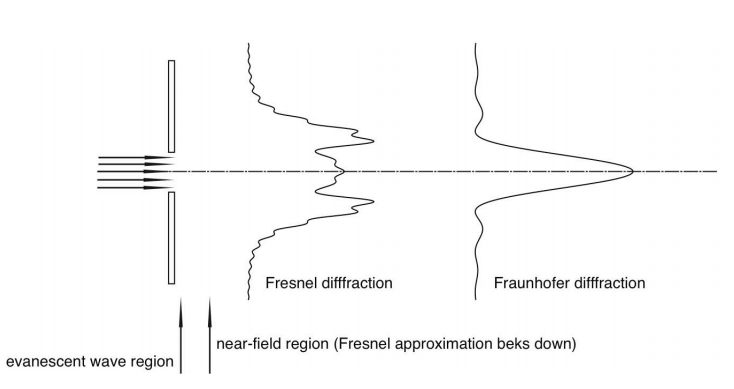# What are Difference between Fresnel and Fraunhofer Diffraction

Distinguish, differentiate, compare and explain what is the difference between Fresnel and Fraunhofer diffraction. Comparison and Differences.

The spreading out of light wave into the geometrical shadow when it passes through a narrow opening is known as the diffraction. The difference between Fresnel and Fraunhofer diffraction is as follows:

## Comparison and difference between Fresnel and Fraunhofer Diffraction

 S.No. Fresnel Diffraction Fraunhofer Diffraction 1 The source and the screen are at a finite distance from the diffracting aperture. The source, and the screen is at an infinite distance from the diffracting aperture. 2 For obtaining Fresnel diffraction, zone plates are used. The single-double plane diffraction grating is used. 3 The wave-fronts are divergent either spherical and cylindrical. The wave-fronts are the plane which is realized by using the convex lens. 4 No mirror or lenses are used for observations. Diffracted light is collected by a lens as in a telescope.

According to Huygens, each point on a wavefront serves as the source of a spherical secondary wavelet with the same frequency as the primary wave. The amplitude at any point is the superposition of these wavelets.

Fraunhofer and Fresnel Diffraction

Consider an opaque screen illuminated with a plane wave. The light spreads as a result of diffraction. If the observation screen is far enough away from the aperture, the diffraction pattern does not change in structure, but merely changes in size, as the distance is further increased. This situation is called Fraunhofer diffraction (shown in the image below).Closer to the aperture the diffraction pattern does change with distance. This is called Fresnel diffraction. Calculation of Fresnel diffraction is based on an approximation, which eventually breaks down: closer to the aperture more advanced theories are required. The Fraunhofer diffraction pattern is obtained at a very large distance from the aperture, but using a lens, an image of it can be formed at a finite distance. In this case, the regions either closer or further from the lens give Fresnel diffraction.

Tags:

Difference between Fraunhofer Diffraction vs Fresnel

Fresnel vs Fraunhofer Diffraction

Differences between Fraunhofer Diffraction vs Fresnel